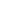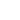AI

# 机器听觉：四、在自编码器架构中加入记忆机制

【编者按】Kanda机器学习工程师Daniel Rothmann撰写的机器听觉系列第四篇，讲解如何在声音频谱嵌入中加入记忆机制。#### 进入循环``````import numpy as np

class CircularBuffer():
# 初始化NumPy数组和头/尾指针
def __init__(self, capacity, dtype=float):
self._buffer = np.zeros(capacity, dtype)
self._tail_index = 0
self._capacity = capacity

# 确保头指针和尾指针循环往复
def fix_indices(self):
self._tail_index -= self._capacity
self._tail_index += self._capacity

# 在缓冲区中插入新值，如缓冲区已满，覆盖旧值
def insert(self, value):
if self.is_full():

self._buffer[self._tail_index % self._capacity] = value
self._tail_index += 1
self.fix_indices()

# 将环形缓冲区作为以头指针为开始的数组返回
def unwrap(self):
return np.concatenate((
self._buffer[:max(self._tail_index - self._capacity, 0)]
))

# 指示缓冲区是否已满
def is_full(self):
return self.count() == self._capacity

# 返回缓冲区中的当前值
def count(self):
``````

#### 降低输入尺寸``````from gammatone import gtgram
import numpy as np

class GammatoneFilterbank:
def __init__(self,
sample_rate,
window_time,
hop_time,
num_filters,
cutoff_low):
self.sample_rate = sample_rate
self.window_time = window_time
self.hop_time = hop_time
self.num_filters = num_filters
self.cutoff_low = cutoff_low

def make_spectrogram(self, audio_samples):
return gtgram.gtgram(audio_samples,
self.sample_rate,
self.window_time,
self.hop_time,
self.num_filters,
self.cutoff_low)

def make_dilated_spectral_frames(self,
audio_samples,
num_frames,
dilation_factor):

spectrogram = self.make_spectrogram(audio_samples)
spectrogram = np.swapaxes(spectrogram, 0, 1)
dilated_frames = np.zeros((len(spectrogram),
num_frames,
len(spectrogram)))

for i in range(len(spectrogram)):
for j in range(num_frames):
dilation = np.power(dilation_factor, j)

if i - dilation < 0:
dilated_frames[i][j] = spectrogram
else:
dilated_frames[i][j] = spectrogram[i - dilation]

return dilated_frames
``````#### 嵌入缓冲区#### 基于Keras

``````from keras.models import Model
from keras.layers import Input, LSTM, RepeatVector

def prepare_listener(timesteps,
input_dim,
latent_dim,
optimizer_type,
loss_type):

inputs = Input(shape=(timesteps, input_dim))

encoded = LSTM(int(input_dim / 2),
activation="relu",
return_sequences=True)(inputs)

encoded = LSTM(latent_dim,
activation="relu",
return_sequences=False)(encoded)

decoded = RepeatVector(timesteps)(encoded)

decoded = LSTM(int(input_dim / 2),
activation="relu",
return_sequences=True)(decoded)

decoded = LSTM(input_dim,
return_sequences=True)(decoded)

autoencoder = Model(inputs, decoded)
encoder = Model(inputs, encoded)

autoencoder.compile(optimizer=optimizer_type,
loss=loss_type,
metrics=['acc'])

return autoencoder, encoder
``````#### 参考文献

1. J. J. Eggermont, “Between sound and perception: reviewing the search for a neural code.,” Hear. Res., vol. 157, no. 1–2, pp. 1–42, Jul. 2001.
2. C. Alain, D. L. Woods, and R. T. Knight, “A distributed cortical network for auditory sensory memory in humans,” Brain Res., vol. 812, no. 1–2, pp. 23–37, Nov. 1998.
3. A. Wingfield, “Evolution of Models of Working Memory and Cognitive Resources,” Ear Hear., vol. 37, p. 35S–43S, 2016.
4. “Implementing Circular/Ring Buffer in Embedded C”, Embedjournal.com, 2014. [Online]. Available: https://embedjournal.com/implementing-circular-buffer-embedded-c/
5. A. Van Den Oord et al., “Wavenet: A Generative Model for Raw Audio.”
6. Y.-A. Chung, C.-C. Wu, C.-H. Shen, H.-Y. Lee, and L.-S. Lee, “Audio Word2Vec: Unsupervised Learning of Audio Segment Representations Using Sequence-to-Sequence Autoencoder,” in Proceedings of the Annual Conference of the International Speech Communication Association, 2016, pp. 765–769.
7. F. Chollet, “A ten-minute introduction to sequence-to-sequence learning in Keras”, Blog.keras.io, 2018. [Online]. Available: https://blog.keras.io/a-ten-minute-introduction-to-sequence-to-sequence-learning-in-keras.html

### 评论游客2017-08-30

2017-11-20

2017-04-19

### 【原创】神经网络架构

2018-01-22发布需求联系客服